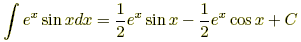# integration by parts

Integration by Parts

A formula used to integrate the product of two functions.

Formula: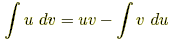Example 1:Evaluate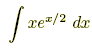.

Use u = x and dv = ex/2 dx. Then we get du = dx and v = 2ex/2. This can be summarized:

 u = x dv = ex/2 dx du = dx v = 2ex/2

It follows that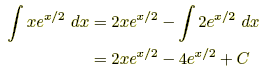Example 2:Evaluate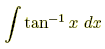.

Use the following:

 u = tan-1x dv = dxv = x

Thus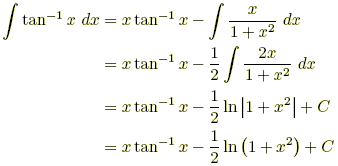Example 3:Evaluate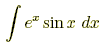.

Let I =. Proceed as follows:

 u = sinx dv = exdx du = cosx dx v = ex

Thus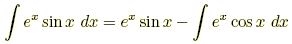Now use integration by parts on the remaining integral . Use the following assignments:

 u = cosx dv = exdx du = –sinx dx v = ex

Thus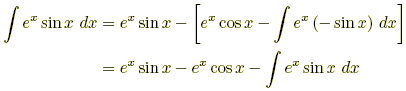Note thatappears on both sides of this equation. Replace it with I and then solve.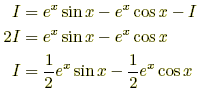We finally obtain